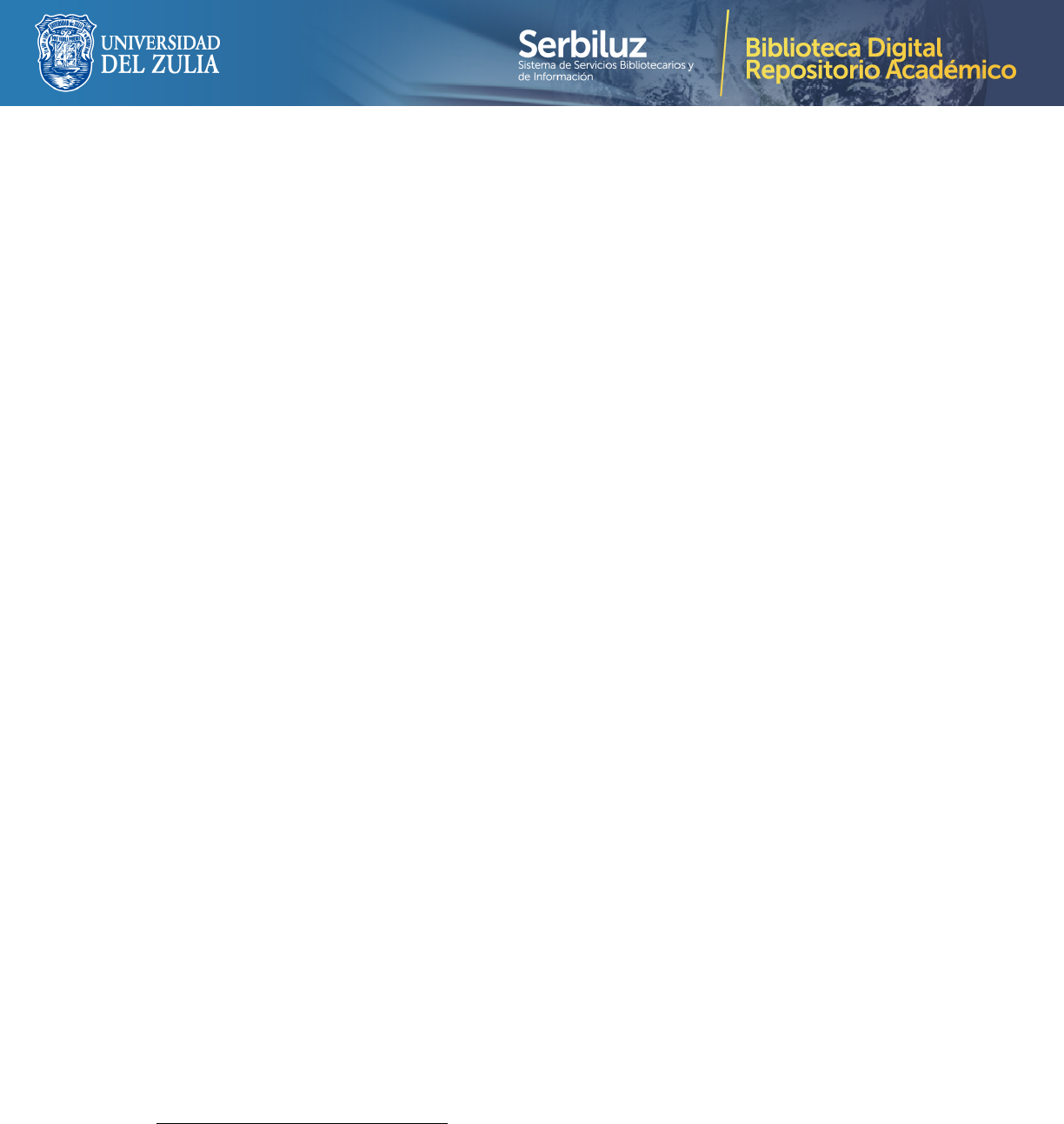Divulgaciones Matem´aticas Vol. 22, No. 1(2021), pp. 1–21
Caputo and Caputo-Fabrizio fractional
ascaras diferenciales fraccionarias de Caputo y Caputo-Fabrizio para la mejora
de im´agenes
Gustavo Asumu MBoro Nchama (asumu@matcom.uh.cu)
Universidad Nacional de Guinea Ecuatorial (UNGE)
Malabo, Guinea Ecuatorial, Calle Hassan II
Leandro Daniel Lau Alfonso (leandro@icimaf.cu)
Instituto de Cibern´etica Matem´atica y F´ısica, ICIMAF
Calle 15, No. 551, entre C y D, Vedado, Habana 4, CP–10400, Cuba
Ana Luisa Morales Galloso (pedrosoaugusto584@gmail.com)
Facultad de Ciencias Medicas, Miguel Enr´ıquez
Ram´on Pinto y Ensenada Luyano, La Habana
Abstract
Image enhancement is one of the most important tasks in the ﬁeld of image processing.
With the help of computer and programming languages many mathematical methods have
been implemented to improve the visual quality of an image. One of the most eﬀective meth-
ods for this purpose is the histogram equalization. The construction of fractional diﬀerential
masks for images enhancement has also been proposed. In this paper, we propose a new
way of construction of fractional diﬀerential mask based on the Caputo and Caputo-Fabrizio
derivatives. The eﬀectiveness of the proposed methods have been compared with the his-
togram equalization method and the multiplication of each pixel of an image by a constant.
The experiments results have shown superiority of the proposed methods, with better visual
quality and higher gray-level co-occurrence matrix values in four directions.
Key words and phrases: Contrast image enhancement, fractional calculus, fractional
Resumen
La mejora de im´agenes es una de las tareas as importantes en el campo del proce-
samiento de im´agenes. Con la ayuda de lenguajes inform´aticos y de programaci´on, se han
implementado muchos etodos matem´aticos para mejorar la calidad visual de una imagen.
Uno de los etodos m´as eﬁcaces para este prop´osito es la ecualizaci´on del histograma. Tam-
bi´en se ha propuesto la construcci´on de ascaras diferenciales fraccionarias para la mejora
de im´agenes. En este art´ıculo, se propone una nueva forma de construcci´on de ascara di-
ferencial fraccional basada en las derivadas de Caputo y Caputo-Fabrizio. La eﬁcacia de los
m´etodos propuestos se ha comparado con el m´etodo de ecualizaci´on del histograma y la
Received 9/12/2020. Revised 12/01/2021. Accepted 11/04/2021.
MSC (2010): Primary 34Axx; Secondary 65Lxx.
Corresponding author: Gustavo Asumu MBoro Nchama2 Gustavo MBoro - Leandro Lau - Ana Morales
multiplicaci´on de cada p´ıxel de una imagen por una constante. Los resultados de los expe-
visual y valores de matriz de co-ocurrencia de nivel de gris as altos en cuatro direcciones.
Palabras y frases clave: Mejora de la imagen de contraste, c´alculo fraccional, M´ascara
diferencial fraccionaria.
1 Introduction
Digital image processing is a set of techniques applied to digital images with the aim of improving
their quality using a computer. For years, these type of techniques have been investigated and
used in applications for diﬀerent tasks such as image enhancement, image restoration and image
edge detection, among others. For the image enhancement, various methods have been proposed:
Histogram Equalization (HE) is one of the best used methods for image enhancement. It is
provides better quality of images without loss of any information . The multiplication of
each pixel of an image by a constant is also one of the eﬀective methods to make image clearer.
Recently, many authors have proposed the construction of masks, for image enhancement, based
on fractional derivatives [38-47]. A fractional derivative is an integral operator which generalizes
the ordinary derivative, such that if the fractional derivative is represented by D
α
then, when
α = n, it coincides with the usual diﬀerential operator D
n
. Such kind of operators are deﬁned
by the help of spaces as:
Deﬁnition 1.1. A function f : [a, b] R is said to be absolutely continuous on [a, b], denoted
by f AC[a, b], if given > 0 there exists some σ > 0 such that
n
X
k=1
|f(y
k
) f(x
k
)| < .
whenever {[x
k
, y
k
] : k = 1, · · · , n} is a ﬁnite collection of mutually disjoint subintervals of [a, b]
with
n
X
k
(y
k
x
k
) < σ.
Deﬁnition 1.2. Let n N and k = 1, 2, · · · , n 1, the space AC
n
[a, b] is deﬁned by
AC
n
[a, b] := {f : [a, b] R : f
(k)
(t) C[a, b], f
(n1)
(t) AC[a, b]}.
There are many deﬁnitions of fractional derivatives [42-4]. One of the most popular was
deﬁned by Gronwald and Letnikov:
Deﬁnition 1.3. Let a, b R with a < b, α > 0, f C
n
[a, b]. The Gronwald-Letnikov (GL)
fractional derivative of order α, is given by
GL
D
α
at
f(t) = lim
h0
+
1
h
α
[
xa
h
]
X
k=0
(1)
k
α
k
f(t kh). (1)
where
xa
h
denotes the integer part of
xa
h
.
Divulgaciones Matem´aticas Vol. 22, No. 1(2021), pp. 1–21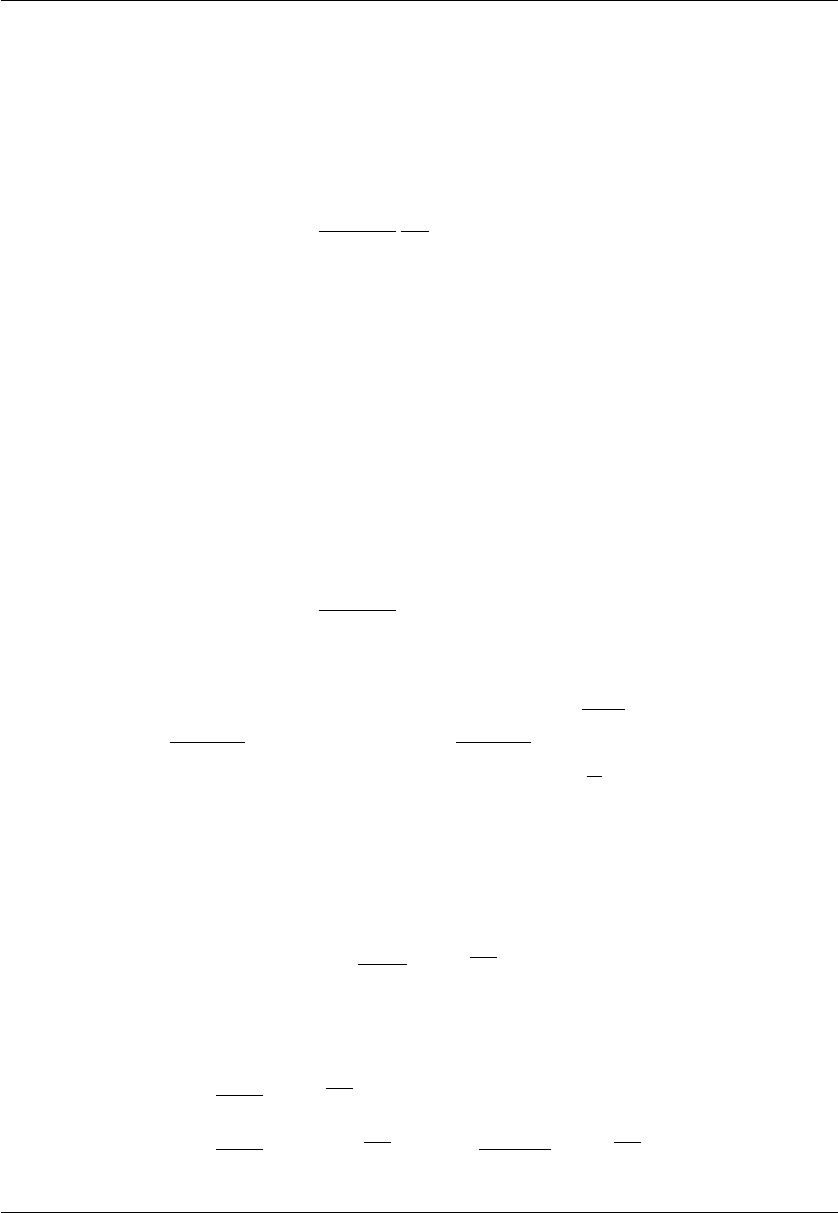Caputo and Caputo-Fabrizio fractional diﬀerential masks for image enhancement 3
One of the inconveniences of Gronwald-Letnikov derivative is that the class of functions for
which this derivative is deﬁned is very narrow. To overcome this inconvenience, Riemann and
Liouville proposed the following deﬁnition :
Deﬁnition 1.4. Let a, b R with a < b, α > 0, f AC
n
[a, b]. The Riemann-Liouville (RL)
fractional derivative of order α, is deﬁned by
RL
D
α
at
f(t) =
1
Γ(n α)
d
n
dt
n
t
Z
a
(t s)
n(α+1)
f(s)ds.
Even though the RL approach overcomes the drawbacks related the GL deﬁnition and it has
been applied successfully in many areas of engineering, unfortunately, it leads to initial conditions
containing the limit values of the RL fractional derivative at the lower terminal t = a, for example
lim
ta
{
RL
D
α1
at
f(t)} = b
1
, lim
ta
{
RL
D
α2
at
f(t)} = b
2
, . . . , lim
ta
{
RL
D
αn
at
f(t)} = b
n
.
In spite of the fact that initial value problems with such initial conditions can be successfully
solved mathematically, their solutions are practically useless, because there is no known physical
interpretation for such types of initial conditions. An alternative solution to this conﬂict was
proposed by M. Caputo :
Deﬁnition 1.5. Let a, b R with a < b, α > 0, f AC
n
[a, b]. The Caputo fractional derivative
of order α, is deﬁned by
C
D
α
at
f(t) =
1
Γ(n α)
t
Z
a
(t s)
n(α+1)
f
(n)
(s)ds. (2)
For 0 < α 1 and a = 0, the numerical approximation of (2) takes the form
C
D
α
0x
u(x) =
1
Γ(1 α)
Z
x
0
(x ξ)
α
u
0
(ξ)
1
Γ(1 α)
N1
X
k=0
(k+1)x
N
Z
kx
N
(x ξ)
α
u
0
(ξ
k
). (3)
To describe material heterogeneity and structures with diﬀerent scales which cannot be well
described by classical local theories or by fractional models with singular kernel, Caputo and
Fabrizio introduced a new fractional approach :
Deﬁnition 1.6. Let a, b R with a < b, 0 < α < 1, f AC
1
[a, b]. The Caputo-Fabrizio
fractional derivative of order α, is deﬁned by
CF
D
α
ax
u(x) =
M(α)
1 α
Z
x
a
e
α
1α
(xs)
u
0
(s)ds,
where M (α) is a function such that M(0) = M(1) = 1.
In , Losada and Nieto, suggested the following particular case
CF
D
α
ax
u(x) =
1
1 α
Z
x
a
e
α
1α
(xs)
u
0
(s)ds (4)
=
1
1 α
u(x) e
α
1α
x
u(a)
α
(1 α)
2
Z
x
a
e
α
1α
(xτ )
u(τ).
Divulgaciones Matem´aticas Vol. 22, No. 1(2021), pp. 1–21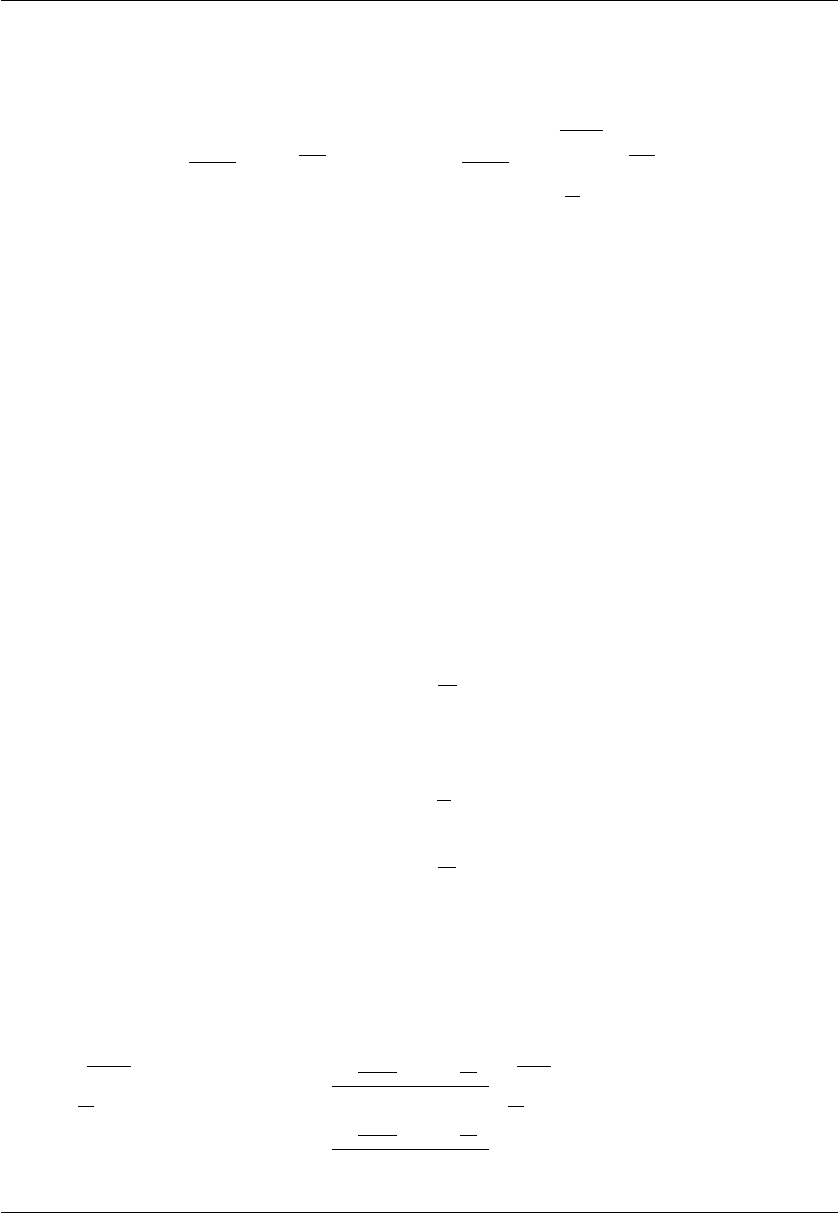4 Gustavo MBoro - Leandro Lau - Ana Morales
Taking a = 0, formula (4) can be approximated as
CF
D
α
0x
u(x) =
1
1 α
Z
x
0
e
α
1α
(xξ)
u
0
(ξ)
1
1 α
N1
X
k=0
(k+1)x
N
Z
kx
N
e
α
1α
(xξ)
u
0
(ξ
k
). (5)
Fractional derivatives provide interesting possibilities for scientiﬁc ﬁelds such as anomalous diﬀu-
sion [40-23], circuit theory [3-43], image processing [33-26] and many others [1-29]. The purpose
of this paper is to improve the visual quality of dark images by using Fractional Diﬀerential
Masks in Caputo (FDMC) and Caputo-Fabrizio (FDMCF) senses. The remainder of this paper
is organized as follows: in section 2, we construct a fractional diﬀerential mask in the Caputo
sense, next, fractional diﬀerential mask in Caputo-Fabrizio sense is given in section 3. Section 4
presents the experimental results of the proposed methods. A conclusion is considered in section
5.
2 Prewitt fractional ﬁlter in the Caputo sense
The goal of this section is to costruct a fractional diﬀerential mask based on the Caputo derivative
deﬁnition. For this purpose, we ﬁrst discretize numerically the Caputo derivative based on the
forward ﬁnite diﬀerence scheme in the interval [0, x] (analogously [0, y]). Let’s take a partition
of N nodes of the interval [0, x], with step x =
x
N
. Thus, there are N + 1 nodes. The N + 1
causal pixels can be given by
u
0
= u(0)
u
1
= u(
x
N
)
.
.
.
u
k
= u(
kx
N
)
.
.
.
u
N
= u(x),
For α (0, 1), by approximating, we obtain
Z
(k+1)x
N
kx
N
(x ξ)
α
u
0
(ξ
k
)
u
kx+x
N
u
kx
N
x
Z
kx+x
N
kx
N
(x ξ)
α
=
u
kx+x
N
u
kx
N
(1 α)(∆x)
α
(N k 1)
1α
(N k)
1α
. (6)
Divulgaciones Matem´aticas Vol. 22, No. 1(2021), pp. 1–21Caputo and Caputo-Fabrizio fractional diﬀerential masks for image enhancement 5
Then, taking (6) into (3), we have
C
D
α
0x
u(x)
1
(∆x)
α
Γ(2 α)
N1
X
k=0

u
(k + 1)x
N
u
kx
N

(N k 1)
1α
(N k)
1α
=
1
(∆x)
α
Γ(2 α)
1
1α
u
N
+
2
1α
2 · 1
1α
u
N1
+
2 · 2
1α
3
1α
1
1α
u
N2
+
(N j 1)
1α
+ (N j + 1)
1α
2(N j)
1α
u
j
+ · · · +
(N 2)
1α
2(N 1)
1α
+ N
1α
u
1
+
(N 1)
1α
N
1α
u
0
. (7)
The anterior approximate diﬀerence of fractional partial diﬀerential on x and y coordinates are
expressed as
C
D
α
0x
u(x, y)
1
(∆x)
α
Γ(2 α)
1
1α
u(x, y) +
2
1α
2 · 1
1α
u(x 1, y)
+
2 · 2
1α
3
1α
1
1α
u(x 2, y)
+ · · · +
(N 1)
1α
N
1α
u(x n, y)
, (8)
and
C
D
α
0y
u(x, y)
1
(∆x)
α
Γ(2 α)
1
1α
u(x, y) +
2
1α
2 · 1
1α
u(x, y 1)
+
2 · 2
1α
3
1α
1
1α
u(x, y 2)
+ · · · +
(N 1)
1α
N
1α
u(x, y n)
. (9)
As in a digital 2-D gray image u(x, y), the shortest distance on x and y coordinates is one pixel,
then we put x = y = 1, and from (7), we obtain N + 1 coeﬃcients c
i
(i = 0, . . . , N), which
Divulgaciones Matem´aticas Vol. 22, No. 1(2021), pp. 1–21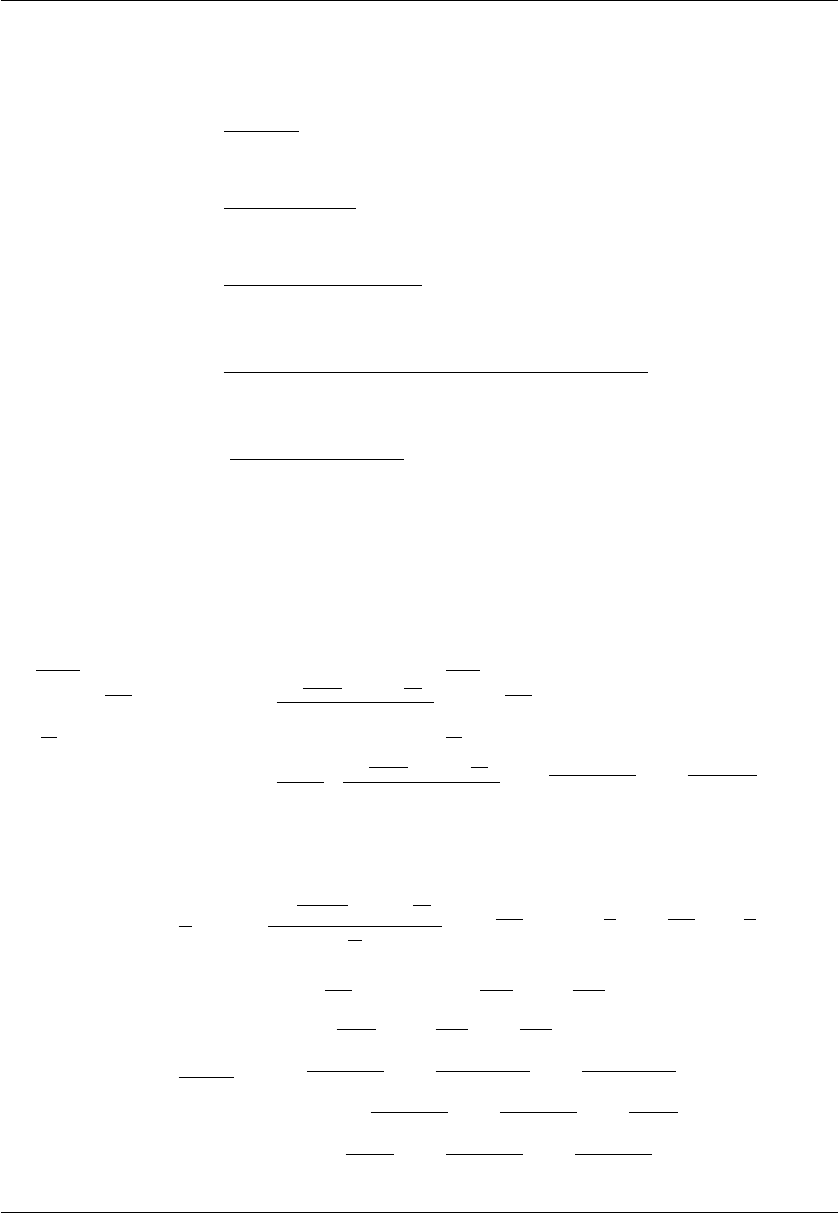6 Gustavo MBoro - Leandro Lau - Ana Morales
depend on the fractional order α:
c
0
=
1
1α
Γ(2 α)
,
c
1
=
2
1α
2 · 1
1α
Γ(2 α)
,
c
2
=
2 · 2
1α
3
1α
1
1α
Γ(2 α)
,
.
.
.
c
j
=
(N j 1)
1α
+ (N j + 1)
1α
2(N j)
1α
Γ(2 α)
,
.
.
.
c
N
=
(N 1)
1α
N
1α
Γ(2 α)
.
3 Prewitt fractional ﬁlter in the Caputo-Fabrizio sense
Following the idea as in the previous section, we obtain
(k+1)x
N
Z
kx
N
e
α
1α
(xξ)
u
0
(ξ
k
)
u
kx+x
N
u
kx
N
x
kx+x
N
Z
kx
N
e
α
1α
(xξ)
,
=
1 α
α
·
u
kx+x
N
u
kx
N
x
·
e
α(Nk1)∆x
1α
e
α(Nk)∆x
1α
. (10)
Inserting (10) into (5), we have
CF
D
α
0x
u(x)
1
α
N1
X
k=0
u
(k+1)x
N
u
kx
N
x
N
e
α
1α
[N(k+1)]
x
N
e
α
1α
[Nk]
x
N
=
1
α · x
1 e
α
1α
x
u
N
+
2e
α·x
1α
e
2
α·x
1α
1
u
N1
+
2e
2α·x
1α
e
3
α·x
1α
e
α·x
1α
u
N2
+ · · · +
2e
α·(Nj)·x
1α
e
α·(Nj1)·x
1α
e
α·(Nj+1)·x
1α
u
j
+ · · · +
2e
α·(N1)·x
1α
e
α·(N2)·x
1α
e
α·N·x
1α
u
1
+
2e
α·N·x
1α
e
α·(N1)·x
1α
e
α·(N+1)·x
1α
u
0
. (11)
Divulgaciones Matem´aticas Vol. 22, No. 1(2021), pp. 1–21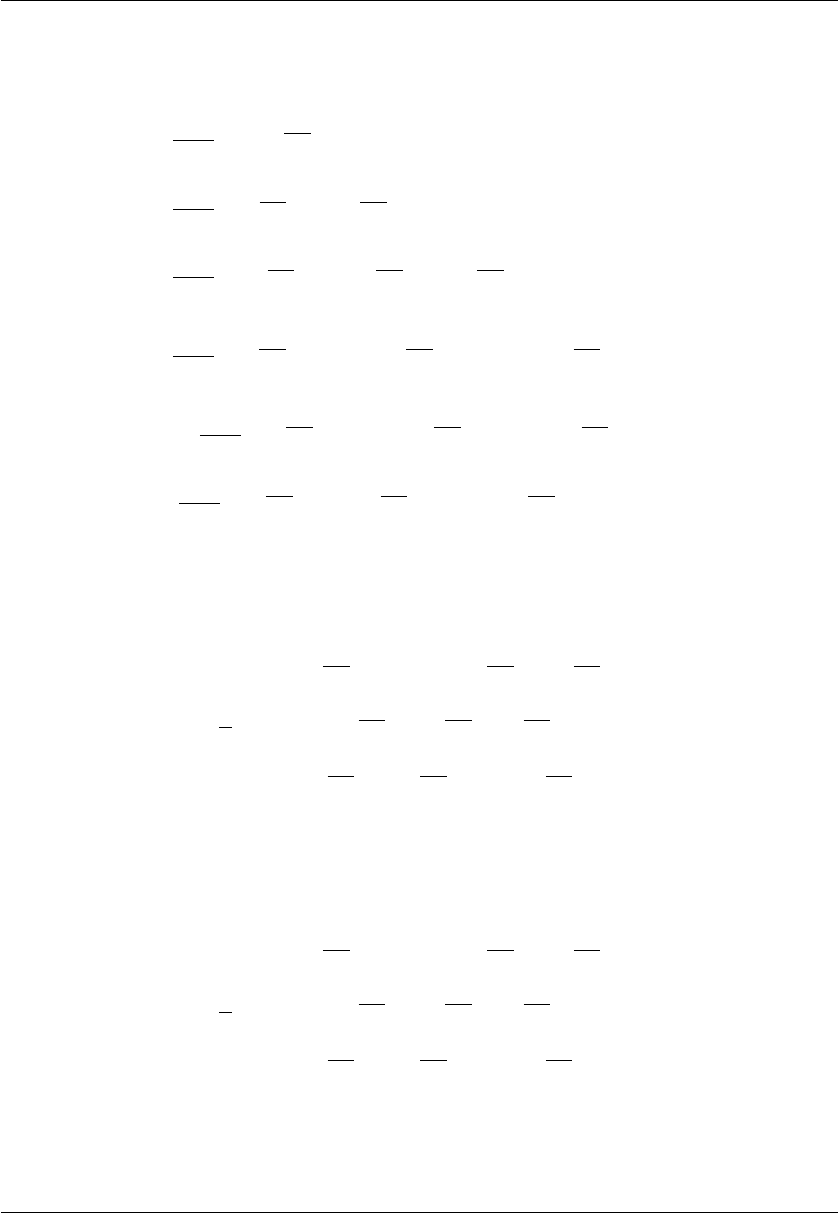Caputo and Caputo-Fabrizio fractional diﬀerential masks for image enhancement 7
From (11), we obtain N + 1 nonzero coeﬃcients c
i
(i = 0, . . . , N), given by
c
0
=
1
αx
1 e
α
1α
x
,
c
1
=
1
αx
2e
α
1α
x
e
2α
1α
x
1
,
c
2
=
1
αx
2e
2
α
1α
x
e
3
α
1α
x
e
α
1α
x
,
.
.
.
c
j
=
1
αx
2e
α
1α
(Nj)∆x
e
α
1α
(Nj1)∆x
e
α
1α
(Nj+1)∆x
,
.
.
.
c
N1
=
1
αx
2e
α
1α
(N1)∆x
e
α
1α
(N2)∆x
e
α
1α
Nx
,
c
N
=
1
αx
2e
α
1α
Nx
e
α
1α
(N1)∆x
e
α
1α
(N+1)∆x
.
Taking x = ∆y = 1, as in the previous section, we obtain the followings two expressions:
CF
D
α
0y
u(x, y)
1
α
1 e
α
1α
u(x, y) +
2e
α
1α
e
2
α
1α
1
u(x 1, y)
+
2e
2
α
1α
e
3
α
1α
e
α
1α
u(x 2, y) + · · ·
+
2e
α
1α
N
e
α
1α
(N1)
e
α
1α
(N+1)
u(x n, y)
, (12)
and
CF
D
α
0y
u(x, y)
1
α
1 e
α
1α
u(x, y) +
2e
α
1α
e
2
α
1α
1
u(x, y 1)
+
2e
2
α
1α
e
3
α
1α
e
α
1α
u(x, y 2) + · · ·
+
2e
α
1α
N
e
α
1α
(N1)
e
α
1α
(N+1)
u(x, y n)
. (13)
The next images show the results of applying the proposed FDMCF, with diﬀerent values of
diﬀerential order α, on the following images: goldhill image, drak bedroom and dark room.
Divulgaciones Matem´aticas Vol. 22, No. 1(2021), pp. 1–21